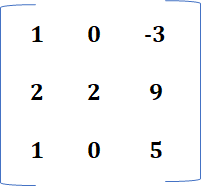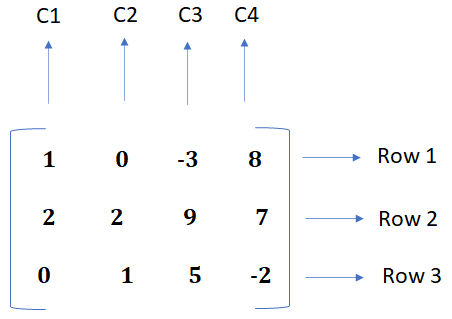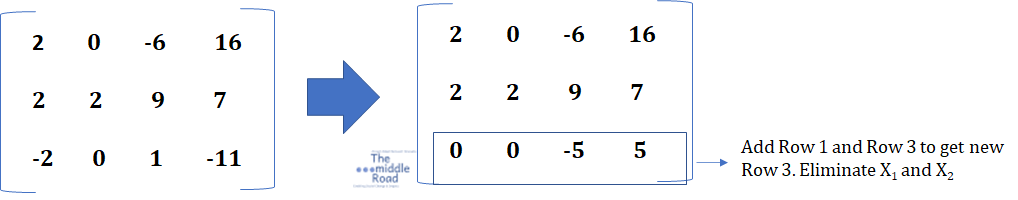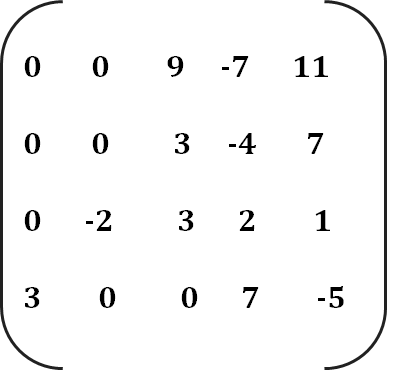# Module 1 : Introduction to Linear Algebra

### This educational video series will include the following.

Introduction to Linear Algebra

Overview of Vectors and Matrix

Solving Linear Equations:

Elimination Method using Matrices

The reference book for this series is Linear Algebra and its Applications by David C Lay. Another book, Linear Algebra and its applications by Gilbert Strang is also recommended. Gilbert Strang also teaches a course on this topic that is freely available at MIT Open Courseware. The first educational video is dedicated to understand techniques to solve systems of linear equations (collection of one or more linear equations having same variables X1 ….. Xn ) the first step in this Linear Algebra course. Two videos include a solved example and this read has one of the problems covered in the video solved step by step in the matrix notation followed by another one. Linear algebra forms the back bone of mathematical modelling example linear programming used in every major industry that range from economics, engineering, space technology to electrical networks. In Linear Algebra usually the solution is given.

Wassily Leontief, won the Nobel Prize in Economic Sciences for his work in input and output analysis. He is the architect behind the mathematical modelling, a pioneer in using computers to analyze these complex  calculations. Feel free to refer to book of your choice but reference to a decent book would be very helpful.  Many resources are on the web free of cost. This course is designed to be quant heavy, supplemented with many problems and solutions to make you competent in this subject.

## Introduction to Linear Algebra

Note in the video D is written as L. Here C denotes the coefficients of the equation and L the solution. C and L can be real or complex numbers while the subscript n can take any positive number from number 2 to that in thousands or more.

C1X1 + C2 X2 + C3X3 + …………………………Cn Xn = D

## # Scalars and Vectors

We start by understanding the difference between scalars and vectors. Scalars give magnitude while vectors give both direction and magnitude. Entropy and speed are examples of scalar while velocity and force examples of vector.  For example traveling in north east at 80 km per hour denotes both magnitude and direction. In vectors arrow symbolizes the direction of action and length the magnitude of the action. A simple statement of 80 km per hour would be the a scalar quantity but giving direction (north east) becomes a vector.

Example: You travel from your house north for 3 km then turn west for 2 km to reach your destination.

3 km = a, 2 km = b are scalar representation.  V = ai+ bj;    here i is a unit vector pointing north,  j is a unit vector pointing west

Matrix with only one column are known as a vector or column vector. Vector will be revisited in the topic r-two. Refer to the tutorial to know more.

A system of linear equations can have any of the three outcomes. It has either one, no, or infinite solutions. Example linear equations that can be plotted in two dimensions; graph of these equations are lines that will interest at one point for a system of linear equations that has a unique solution or be parallel for those systems that have no solution. A good way of identifying equations that have no solution is when the coefficients of the set of equations are zero. This means that when in any row in a matrix, if the coefficients are zero, then the matrix has no solution. Systems with no solution are inconsistent.

Equations that have infinite solutions have lines that overlap or coincide with each other, hence many solutions. The elimination method is used to calculate the solution for systems of linear equations, a technique used by algorithms in computers to calculate solutions for complex systems. A system of linear equations is consistent if it has either a unique or infinite number of solutions. A solution set for a system includes all numbers that are a solution to the set of linear equations within the system. If your linear system has three equations with three variables then the solution set must hold for all three equations. Refer to the module.

### # Linear Equation in Matrix Form

X1 – 3 X3 = 8

2 X1 + 2 + 9 X3 = 7

X2 + 5 X3 = -2Take the below-mentioned example wherein there are three linear equations. On the left side, you have the notation of thelinear equation with all coefficients listed for the respective equations. The first equation X1 – 3X3 = 8  coefficients are 1, 0, and -3, 0 for X2Notice in equation 1 there is no X2 variable implying that the coefficient of the variable is 0. In the first equation, 8 is the constant or the solution of the equation. The augmented matrix consists of all constants of the set of linear equations in the system. Right augmented matrix.

The above system is solved below. The solution set of the system means that the values of the coefficients of X1, X2 ,  Xmust satisfy solutions of all the equations i.e. when substituted they must be equal to the constant on the right hand side of the equation.

The rows of the matrix can be changed interchangeably. Above row2 can be shifted to row3 and vice versa without changing the fundamental properties of the systems of linear equations meaning changing rows has no impact on the result.

### # Solving a Linear System. In this example three linear equation comprise

Below is a solved question from the book Linear Algebra and its Applications by David C. Lay. Solve the systems below and list the solution set.

X1 – 3 X3 = 8

2 X1 + 2 X2 + 9 X3 = 7

X2 + 5 X3 = -2

The following systems equation is written in the matrix form below. There are four columns and three rows and the systems is represented as Matrix A.The above system is represented in the left in the Matrix notation. (Side) The elements here refers to the coefficients of the variables along with their constants. Matrix A is in the augmented matrix (3*4) i.e. 3 rows and 4 columns. (m*n) We need to solve for three variables X1, X2, X3. We can see coefficients of the three variables on the left and the constants on the right side, column C4. To find the solution set for this system of linear equations, use elimination method to find the answer to one variable. Once you have value for any of the variables, use substitution method to find the other solutions.

Looking at the equations in the matrix form, you can see that we can eliminate X2 if we multiply all elements in the Row 3 by 2 and substracting the new equation from that of Row 2. Keeping the equation in the matrix form, multiply Row 3 by 2. Now subtract the new elements of Row 3 from Row 2 to have new elements in Row 3.Now multiply Row 1 by 2 and add Row 1 and Row 3 to get new elements for Row 3. We want to  remove coefficients of two elements or variables so we can find the value of one of the variables. Here by adding Row 1 and Row 3, we remove X1 and  X2  to find the value of  X3.From Row 3,   -5 X3 =  5 which is   X3 = 5/-5 = -1

Substitute the value of  X3 in the system equations given

X2 + 5 X3 = -2               =====  >           X2 + 5(-1) = -2; X2 = -2 +5 = 3

Now we have the following values.  X3 = -1, X2 = 5

Substitute the value of X3 in the below equation to find the value of X1

X1 – 3 X3 = 8     X1 = 8 + 3X3                                                                  =================>                                                           X1 = 8 + 3(-1) = 8-3= 5

### X1= 5; X2 = 3 and  X3 = -1                                                                     Solution set  (5,3-1)

The video below explains the solution to the above system of linear equations in more detail.

Problem Check if the following equation is consistent? Question David C Lay

X1 + 3 X3 = 2

X2 - 3 X4 = 3

-2 X1 + 3 X3 + 2 X4 = 1

3 X1 +7 X4 = -5

First, use the matrix notation for the above problem.There are four variables and one constant, this is a 4* 5 matrix

You need to eliminate three variables to get a solution for one variable. Multiply row 1 by 3 and substract row 4 from row 1.X1 + 3 X3 = 2    *  3      =  3 X1 + 9 X3 = 6

3 X1 + 9 X3 = 6

-    (3 X1 +7 X4 = -5)    =  9 X3 – 7 X4 = 11

Now add row 2 and row 3 for new values for row 2. We use matrix form only to solve the equation. Updating new values for row  2.X2 - 3 X4

+ (- 2 X2 + 3 X3 + 2 X4 = 1)

=======>       3 X3  - 4 X= 7           Row 2

Scale up row 2 by 3.   (3X3  - 4X= 7) * 3   =   9 X3  - 12 X= 21,Now substract this equation from eqa 1 to get new equation for row 1

9 X3 – 7 X4 = 11

-(9 X3  - 12 X= 21)     =============>  5 X4  =  -10

The system of linear equations is now the following

5 X4 = 2

9 X3 – 12 X4 = 21

-2 X2 + 3 X3 + 2 X4 = 1

3 X1 +7 X4 = -5

The first equation reduces to only one variable and now we can find the value of X4

X4 = 10/5 = - 2

Substitute X4 value in 3 X1 +7 X4 = -5

3 X1 +7 (-2) = -5 ====> 3 X1 – 14 = -5

3 X1 = 9    X= 9/3 = 3

You can solve for other variables. Clearly the system is consistent and has a unique solution